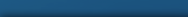# Additional Influencing Factors Affecting The Google PageRank

Many people have discussed the possibility that things beyond the link structure of the web are considered by the PageRank algorithm ever since Page and Brin published the science behind the rankings. In his patent specs, Page discusses a few additional influencing factors. These include:

• Date of link publication
• Significance of the link
• Distance between web pages
• Visibility of the link

The reason additional factors such as these are considered in the PageRank algorithm is the fact that including them leads to a significantly better approximation of human usage deviating from the random surfer model. Considering visibility, position, and significance of the link all allow a better approximation to reality than does the rather static and uninnovative models that require the person to effectively operate as an agent that has no interaction with the website beyond randomly selecting a link on the page.

Of course, these factors were just suggestions on Page’s part, and it’s impossible to run tests to determine whether or not they’ve been incorporated into the Google PageRank algorithm. Because of that, there’s no point in trying to produce a model to replicate behavior that these factors would introduce. Rather, we’ll take a look at how additional influencing factors could be brought into the model, which will reveal quite a bit about how much flexibility Google has in influencing PageRank information after the fact.

## Extending PageRank

Implementing the PageRank algorithm by adding additional ranking factors requires us to modify the algorithm we proposed in the beginning. We can safely make two assumptions: That the PageRank calculations are iterative, and that the number of database queries made during the iterations is minimized for the sake of saving computational time and resources. With that in mind, let’s look at the following extension of the PageRank algorithm:

PR(A) = (1-d) + d (PR(T1)×L(T1,A) + … + PR(Tn)×L(Tn,A))

This looks much the same as before, but with one new addition. The function L(x, y) returns the evaluation of a page x that points to page y. This takes into consideration the number of links between the two, outbound links from each page, and inbound links to each page. This allows for a very holistic evaluation of websites that is organic enough to reflect human tendencies, but mechanical enough to be easily automated.

## Different Link Evaluations

Page mentions visibility and positions of links as two influencing factors. This is sensible, because the visibility and position of a link are obviously huge influencing factors in how frequently a surfer actually clicks them. It’s impossible to have a truly random human surfer: A hidden link will never be followed, and a link at the bottom of a page that has to be scrolled to to be seen will, similarly, be followed less frequently than links towards the top of the page. It’s rather difficult to evaluate PageRank as a function of positions coded in CSS and HTML, so instead, we’ll look at a model that assigns a probability of being followed to each link on the page.

One way to create such a model is to imagine a website with pages A, B, and C, all of which are linked to each other. The idea of probabilities can be realized by assigning integer values that scale with visibilities and positions that make the link easier to see. We might assign a link from B to A a value of 3 if it is present at the top of a page and is bold. Let’s assign probabilities to these sites that allow us to write the following equations:

X(A,B) × Y(A,B) = 1 × 3 = 3

X(A,C) × Y(A,C) = 1 × 1 = 1

X(B,A) × Y(B,A) = 2 × 3 = 6

X(B,C) × Y(B,C) = 2 × 1 = 2

X(C,A) × Y(C,A) = 2 × 3 = 6

X(C,B) × Y(C,B) = 2 × 1 = 2

Because we have to consider the total number of evaluated links on the page as opposed to just the links on a particular page, we must write these weighted quotients:

Z(A) = X(A,B) × Y(A,B) + X(A,C) × Y(A,C) = 4

Z(B) = X(B,A) × Y(B,A) + X(B,C) × Y(B,C) = 8

Z(C) = X(C,A) × Y(C,A) + X(C,B) × Y(C,B) = 8

The values of our L(,) equations work out to the following:

L(A,B) = 0.75

L(A,C) = 0.25

L(B,A) = 0.75

L(B,C) = 0.25

L(C,A) = 0.75

L(C,B) = 0.25

With our standard d = 0.5, we get:

PR(A) = 0.5 + 0.5 (0.75 PR(B) + O.75 PR(C))

PR(B) = 0.5 + 0.5 (0.75 PR(A) + 0.25 PR(C))

PR(C) = 0.5 + 0.5 (0.25 PR(A) + 0.25 PR(B))

. . . Which gives us:

PR(A) = 819/693

PR(B) = 721/693

PR(C) = 539/693

Whew! That was a lot of math. But, there’s definitely a lot to learn from the conclusions. Let’s take a look. First, we can see that page A has the highest rank by far. This is because A receives a more highly rated link from B and C than B and C do from each other. Another interesting fact is that the sum of the PageRanks equals 3 (2079/693). In other words, the sum of the PRs matches the total number of pages. This allows us to do away with the need for normalization and just consider the pages of the sites under consideration. This allows us to minimize the amount of work that needs to be done in ranking and focus instead on dealing with the page rankings, rather than generating them, a wonderful boon for anyone involved in development.

### Related Articles